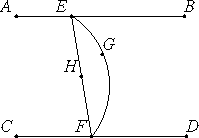# Proposition 7

If two straight lines are parallel and points are taken at random on each of them, then the straight line joining the points is in the same plane with the parallel straight lines.

Let AB and CD be two parallel straight lines, and let points E and F be taken at random on them respectively.I say that the straight line joining the points E and F lies in the same plane with the parallel straight lines.

XI.3

For suppose it is not, but, if possible, let it be in a more elevated plane as EGF. Draw a plane through EGF. Its intersection with the plane of reference is a straight line. Let it be EF.

Therefore the two straight lines EGF and EF enclose an area, which is impossible. Therefore the straight line joined from E to F is not in a plane more elevated. Therefore the straight line joined from E to F lies in the plane through the parallel straight lines AB and CD.

Therefore, if two straight lines are parallel and points are taken at random on each of them, then the straight line joining the points is in the same plane with the parallel straight lines.

Q.E.D.

## Guide

The existence of this proposition is a good argument that Euclid’s definition I.Def.7 of a plane (it lies evenly with the straight lines on itself) does not mean that if two points lie in a plane, then the line joining them also lies in the plane. If it did, then this proposition would be true by definition, and no proof would be required at all.

Note that this proof assumes that every line lies in a plane, a conclusion that has not been justified.

#### Use of this proposition

This proposition is used in the proof of the next as well as proposition XII.17.